# Lines and Angles Questions and Answers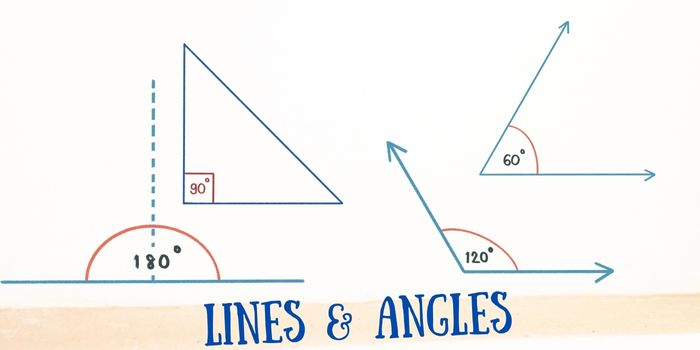## Quiz of Lines and Angles:

Question: If two straight lines have no points in common, they are
(a) always parallel
(b) sometimes parallel
(c) never parallel
(d) always perpendicular

Ans. (a)

Question: In the figure, if PQ PS, PQ || SR, SQR = 35° and UR is the bisector of QRT, URT = 38°, then (x, y) is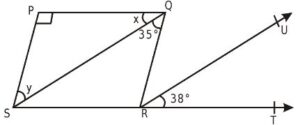(a)  (42°, 48°)
(b) (41°, 49°)
(c) (49°, 41°)
(d) (48°, 42°)

Ans. (b)

Question: Consider the following statements:
(i) If two adjacent angles are equal, then each angle measures 90°
(ii) If the angles forming a linear pair are equal, then each of these angles is of measure  90°
(iii) If two lines intersect and if one pair of vertically opposite angles is formed by acute angles, then another pair of vertically opposite angles will be formed by obtuse angles.
(iv) Angles forming a linear pair. Both are acute angles. The correct option is:
(a) (i), (ii), (iv) are correct
(b) (ii), (iii), (iv) are correct
(c) (i) and (iv) are false
(d) (i) and (iii) are false

Ans. (c)

Question: In the below  figure, ABCD, ABP = 50° and BDC = 50°, then AOD =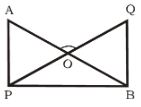(a) 80°
(b) 90°
(c) 100°
(d) 110°

Ans. (d)

Question: The conclusion in the converse of the theorem “If two lines are perpendicular to the same line, they are parallel to each other” is
(a) Two lines are neither parallel nor perpendicular
(b) Two lines are parallel to each other
(c) Two lines are perpendicular to the same line
(d) None

Ans. (c)

Question: In the adjoining figure, a||b||c and l||m, then which of the following is correct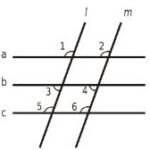(a)  1 = 3
(b) 2 = 3
(c) 4 = 5
(d) 1 = 6

Ans. (d)

Question: Two angles are called adjacent, if
(a) They lie in the same plane and have a cammon vertex
(b) They have a ray in common
(c) The intersection of their interiors is empty
(d) All the above

Ans. (d)

Question: In figure, a is greater than b by one third of a right angle. Then the value of a is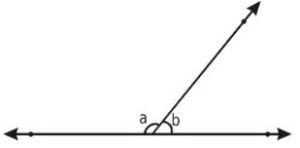(a) 105°
(b) 75°
(c) 135°
(d) 85°

Ans. (a)

Related: Euclidean Geometry Quiz

Question: Consider the following statements:
(i)  A line is that which has length and breadth only.
(ii) A point is that which has no part.
(iii) A line has length but no breadth.
(iv) A plane has no boundaries.
Of these statements which is / are not true.
(a) (i)
(b) Both (ii) and (iv)
(c) (iv)
(d) Both (i) and (iv)

Ans. (a)

Question: In the following figure, l ||m  and p is a transversal, then (x, y) is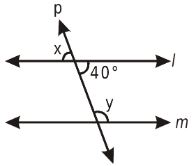(a) (40°, 140°)
(b) (80°, 100°)
(c) (140°, 40°)
(d) (90°, 90°)

Ans. (a)

Question: The set, which contains the intersection points of two non-parallel lines in a plane, is a
(a) Null set
(b) Singleton set
(c) Infinite set
(d) none

Ans. (b)

Question: In the figure, k is equal to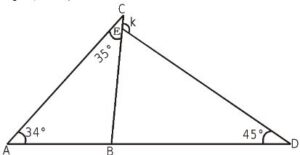(a) 124°
(b) 104°
(c) 114°
(d) 100°

Ans. (c)

Related: questions on mean median mode

Question: Consider the following statements:
(i)  Angles forming a linear pair are supplementary.
(ii) If two adjacent angles are equal, then each angle measures 90°.
(iii) Two distinct lines in a plane cannot have two points in common.
(iv) If the angles forming a linear pair are equal, then each of these angles is of measure 90°
Of these statements, the true statement/s is/ are
(a) Both (ii) and (iii)
(b) Only (ii)
(c) Only (iv)
(d) (i), (iii) and (iv)

Ans. (d)

Question: In the following figure, PQ is an incident ray and QR is the reflected ray. If PQR = 124°, then RQB is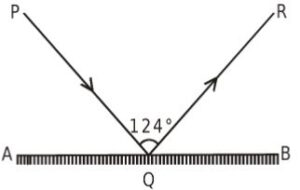(a)  62°
(b) 34°
(c) 56°
(d) 28°

Ans. (d)

Question: Consider the following statements:
(i)  Two planes intersect at a point.
(ii) Two planes intersect in a plane.
(iii) Two lines intersect at a point.
(iv) Two planes intersect in a line.

Of these statements, the false statement/s is /are
(a) Both (i) and (ii)
(b) Both (iii) and (iv)
(c) (i), (ii) and (iii)
(d) only (ii)

Ans. (a)

Question: In the following figure, it is given that AB || CD, BAO = 108° and OCD = 120°. Then AOC is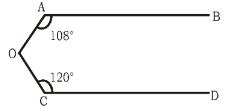(a) 228°
(b) 90°
(c) 72°
(d) 132°

Ans. (d)

Related: area of parallelogram worksheet

Question: The angle which equals two-thirds of its complement is
(a) 72°
(b) 18°
(c) 54°
(d) 36°

Ans. (d)

Question: In the below figure, If PQRS is a parallelogram, then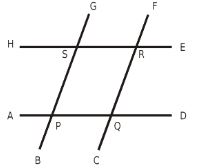(a) APS and HSG are supplementary
(b) GSR and PQR are equal
(c) BPD and ERF are equal
(d) APS and QRS are supplementary

Ans. (d)

Question: In the below  figure, ABCDEFGH is a regular octogon, GDHC and FBC = 60°, then HOF =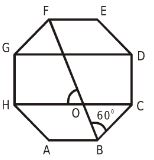(a) 75°
(b) 70°
(c) 60°
(d) 50°

Ans. (c)

Question: The number of angles, formed by a transversal cutting two lines, is
(a) 4
(b) 6
(c) 8
(d) 10

Ans. (c)

Question: In the figure below, ABCE is an Isosceless trapezium, ABDC and BAD = 50°, then DCE =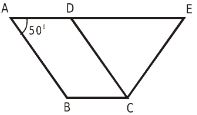(a)  100°
(b)  90°
(c)  80°
(d)  70°

Ans. (a)

Question: In the given figure, PQ || RS, MXQ = 135° and MYR = 40°, then XMY is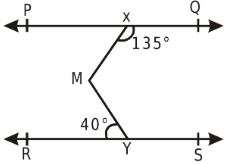(a) 85°
(b) 45°
(c) 180°
(d) 40°

Ans. (a)

Question: In the figure below, ABCD is a square and PQAC,  then PQC =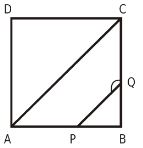(a) 135°
(b) 120°
(c) 145°
(d) 150°

Ans. (a)

Question: Lines PQ and RS intersect at O. If POS = 2 SOQ, then the four angles at O are
(a) 30°, 30°, 120°, 180°
(b) 60°, 60°, 120°, 120°
(c) 60°, 90°, 90°, 120°
(d) 30°, 60°, 90°, 180°

Ans. (b)

Related: square root mathematics quiz

Question: In the figure below, ABCD is a rectangle and BPC and DCQ are equilateral triangles, then PCQ =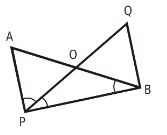(a) 120
(b) 135
(c) 150
(d) 165

Ans. (b)

Question: Consider the following statements relating to 3 lines L1, L2 and L2 in the same plane.
(a) If L2 and L3 are both parallel to L1, then they are parallel to each other.
(b) If L2  and L3 are both perpendicular to L1, then they are parallel to each other.
(c) If the acute angle between L1 and L2 is equal to the acute angle between L1 and L3 then L2 is parallel to L3 of these statements.
(a) 1 and 2 are correct
(b) 1 and 3 are correct
(c) 2 and 3 are correct
(d) 1, 2 and 3 are correct

Ans. (d)

Question: In the below figure, ABCD is a parallelogram, DAB = 140° and FAB = ½ DAB, then FEC =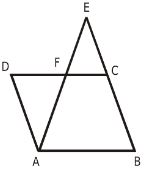(a) 60°
(b) 70°
(c)  75°
(d)  80°

Ans. (c)